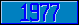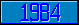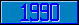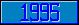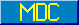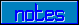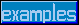## \$%PRODUCE

### M[UMPS] by Example

```
2-Jul–96, 6:54:35

Routine Save for Substring Replacement Library function \$%PRODUCE^STRING

;

; Unless otherwise noted, the code below

; was approved in document X11/95–111

;

; If corrections have been applied,

; first the original line appears,

; with three semicolons at the beginning of the line.

;

; Then the source of the correction is acknowledged,

; then the corrected line appears, followed by a

; line containing three semicolons.

;

PRODUCE(IN,SPEC,MAX) ;

NEW VALUE,AGAIN,P1,P2,I,COUNT

SET VALUE=IN,COUNT=0

FOR  DO  QUIT:'AGAIN

. SET AGAIN=0

. SET I=""

. FOR  SET I=\$ORDER(SPEC(I)) QUIT:I=""  DO  QUIT:COUNT<0

. . QUIT:\$GET(SPEC(I,1))=""

. . QUIT:'(\$DATA(SPEC(I,2))#2)

. . FOR  QUIT:VALUE'[SPEC(I,1)  DO  QUIT:COUNT<0

. . . SET P1=\$PIECE(VALUE,SPEC(I,1),1)

. . . SET P2=\$PIECE(VALUE,SPEC(I,1),2,\$LENGTH(VALUE))

. . . SET VALUE=P1_SPEC(I,2)_P2,AGAIN=1

. . . SET COUNT=COUNT+1

. . . IF \$DATA(MAX),COUNT>MAX SET COUNT=–1,AGAIN=0

. . . QUIT

. . QUIT

. QUIT

QUIT VALUE

;===

;

;

;

```